# 2D FT-ICR¶

This file presents the result of the processing of th 2D FT-ICR data-set.

## Initial remarks¶

2D FT-ICR produces BIG files and generate large computer burden.

FT processing of these large FTICR 2D data-set is out of the scope of this little demo, however, the raw data-set is provided as a csv file.

Two pre-processed data-sets are provided

• a classicaly procesed spectrum
• a spectrum on which rQRd was applied on all the columns after the horizontal FT, and before the vertical FT.

All spectra are presented in modulus. All computations were performed in double precision. However, in order to reduce the file size and the memory burden, the csv files were stored with only single precision.

Even displaying the pre-processed results is quite involving. If you want to give it a try, you will need some time and disk-space.

• 8Mb of central memory is a minimum.
• 2 Gb of disk-space
• a good graphic card

## 1) Defining utility functions¶

In :
%pylab inline
# These functions are utilities used for the processing
def load_csv(fname, si1, si2):
"""
load a file in csv format - knowing the size before hand allows much more efficient loading

to reduce the memory burden the file are loaded in single precision.
reads plain and .gz files
"""
import gzip
import time
print 'please wait...'
t0 = time.time()
d = zeros((si1,si2), dtype=float32)
if fname.endswith('.gz'):
F = gzip.open(fname)
else:
F = open(fname)
i1 = 0         # count rows
for l in F:
if not(l.startswith('#')):
val = array( l.split(','),  dtype=float32)
d[i1, :] = val[:]
i1 += 1
#if i1%50 == 0: print i1
F.close()
print "Done, dataset : %d x %d"%d.shape
print 'loaded in %.0f sec'%(time.time()-t0)
return d

def display_f(d, ax1, ax2, scale=1.0, zoom=None, text=None, mz=None):
"""
Display data in frequency of m/z as a contour plot
scale : determines contour levels
zoom : if present, a tuple defining zoom window ((F1_limits),(F2_limits))

if mz, in m/z units, otherwise in freq
ax1 ax2 are dumb objects containing axes parameters
"""
figure()
(si1,si2) = d.shape
if zoom:        # should ((F1_limits),(F2_limits))
z1lo=zoom
z1up=zoom
z2lo=zoom
z2up=zoom
else:
z1lo=0
z1up=si1-1
z2lo=0
z2up=si2-1
m = abs(d).max()/scale
level = (m*0.5, m*0.25, m*0.1, m*0.05)
step1, step2 = (1,1)
while (z1up-z1lo)/step1 > 2100:    # reduce size of image to 2k x 4k - depends on your graphic card.
step1 *= 2
while (z2up-z2lo)/step2 > 4100:
step2 *= 2
if mz:
axis1 = fticr_mass_axis(ax1)[z1lo:z1up:step1]
axis2 = fticr_mass_axis(ax2)[z2lo:z2up:step2]
else:
axis1 = linspace(0,ax1.freq,si1)[z1lo:z1up:step1]
axis2 = linspace(0,ax2.freq,si2)[z2lo:z2up:step2]
contour(axis2,axis1,d[z1lo:z1up:step1,z2lo:z2up:step2], level)
if text:
title(text)

def fticr_mass_axis(ax):
"""    returns an array which will calibrate a FT-ICR experiment",
ax object with the parameters of the axis
"""
r = arange(ax.length)
r = 1     # as we divide by r, ths part of the axis is not used
r += ax.offset
hz =   r*ax.sw / (ax.length+ax.offset-1)
mz =  ax.mass*ax.freq/hz
return mz
class axis(object):
"will be used as a simple holder for axes"
pass

Welcome to pylab, a matplotlib-based Python environment [backend: module://IPython.zmq.pylab.backend_inline].
For more information, type 'help(pylab)'.

## 2) loading classic spectrum¶

First load the classically processed dataset as d - provided as a csv file

Parameters are as follows :

• Axis F1 : FT-ICR axis at 394.39016 kHz, 2048 real points, from mz = 1000.000 to m/z = 577.561 M/DeltaM (M=400) = 2956
• Axis F2 : FT-ICR axis at 1000.00000 kHz, 131072 real points, from mz = 1000.000 to m/z = 144.390 M/DeltaM (M=400) = 47313
In :
d = load_csv('Datasets/TGplasmahumainNT_2D_processed.csv.gz', si1=2048, si2=128*1024)
ax2 = axis()
ax2.sw = 1000000.0   # spectral width
ax2.freq = 419620    # ref frequency
ax2.mass = 344.0974  # ref mass
ax2.offset = 0       # in points, eventually truncated axis
ax2.length = 128*1024
ax1 = axis()
ax1.sw = 394390.16
ax1.freq = 419620.0
ax1.mass = 344.0974
ax1.offset = 1182
ax1.length = 2048

please wait...
Done, dataset : 2048 x 131072
loaded in 107 sec

## 3) loading the cleaned spectrum¶

And now the urQRd processed data-set as dr

In :
dr = load_csv('Datasets/TGplasmahumainNT_2D_rQRd_processed.csv.gz', si1=2048, si2=128*1024)

please wait...
Done, dataset : 2048 x 131072
loaded in 106 sec

## 4) displaying spectra¶

We'll display then in 3 successive zoom, from the whole spectrum to the detail of the isotopic pattern

First the classic spectrum

In :
display_f(d, ax1, ax2, scale=300, text='classic whole spectrum')
display_f(d, ax1, ax2, zoom=((0,600), (20000,45000)), scale=400, text='classic region of interest')
display_f(d, ax1, ax2, zoom=((50,350), (30000,32000)), scale=600, text='classic details of isotopic patterns')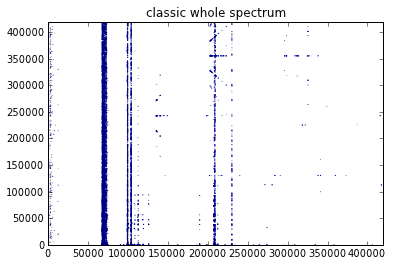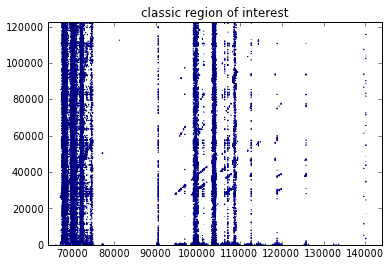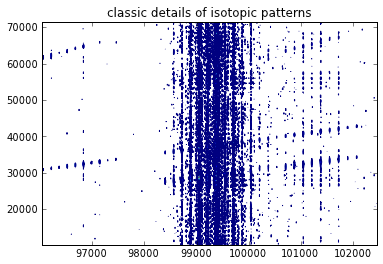Then the cleaned one

In :
display_f(dr, ax1, ax2, scale=600, text='cleaned whole spectrum')
display_f(dr, ax1, ax2, zoom=((0,600), (20000,45000)), scale=400, text='cleaned region of interest')
display_f(dr, ax1, ax2, zoom=((50,350), (30000,32000)), scale=600, text='cleaned details of isotopic patterns')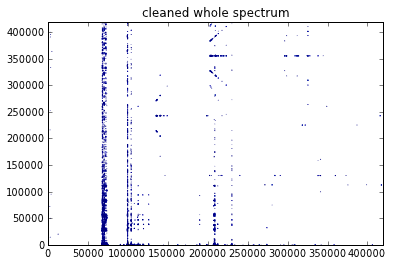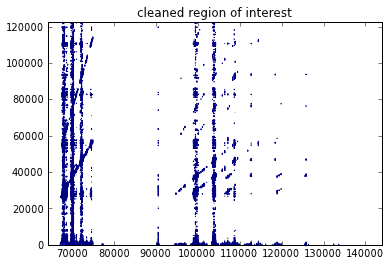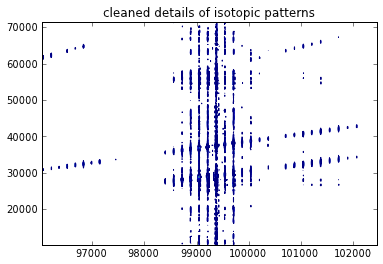Finally in compare both in m/z

In :
display_f(d, ax1, ax2, mz=True, zoom=((100,600), (20000,45000)), scale=400, text='classic region of interest')
display_f(dr, ax1, ax2, mz=True, zoom=((100,600), (20000,45000)), scale=400, text='cleaned region of interest')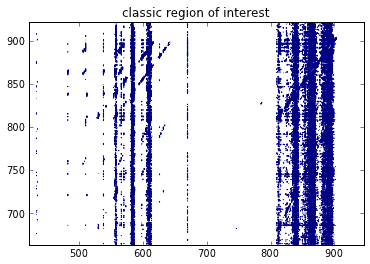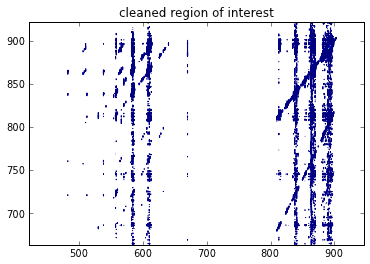In [ ]: